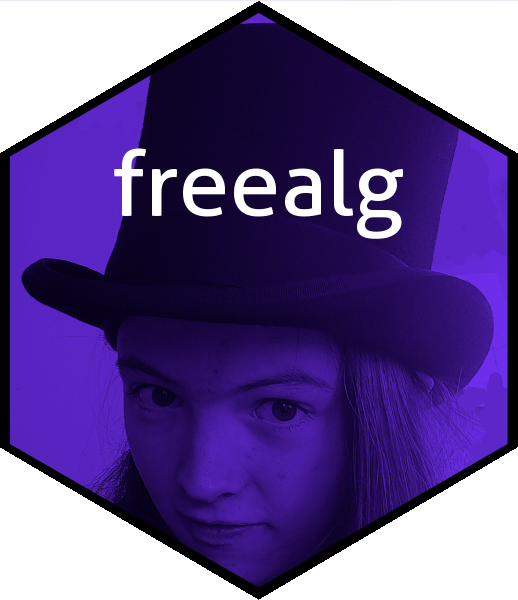# The free algebra with RTo cite the freealg package in publications, please use Hankin (2022b).

The free algebra is best defined by an example: with an alphabet of $$\{x,y,z\}$$, and real numbers $$\alpha,\beta,\gamma$$ we formally define $$A=\alpha x^2yx + \beta zy$$ and $$B=-\beta zy + \gamma y^4$$. Addition is commutative so $$x+y=y+x$$ (and so $$A=\beta zy + \alpha x^2yx$$) but multiplication is not commutative, so $$xy\neq yx$$; both are associative. We also have consistency in that $$\alpha(\beta P)=(\alpha\beta)P$$ for any expression $$P$$. Then:

$A+B=(\alpha x^2yx + \beta zy) + (-\beta zy + \gamma y^4) = \alpha x^2yx + \gamma y^4$

$AB= (\alpha x^2yx + \beta zy) (-\beta zy + \gamma y^4) = -\alpha\beta x^2yxzy +\alpha\gamma x^2yxy^4 -\beta^2zyzy +\beta\gamma zy^5$

$BA=(-\beta zy + \gamma y^4)(\alpha x^2yx + \beta zy) = -\alpha\beta zyx^2yx -\beta^2 zyzy + \alpha\gamma y^4x^2yx + \beta\gamma y^4zy$

This is a natural set of objects to consider. Formally, we consider the free R-module with a basis consisting of all words over an alphabet of symbols [conventionally lower-case letters] with multiplication of words defined as concatenation. The system inherits associativity from associativity of concatenation; distributivity follows from the definition of R-module. However, the free algebra is not commutative in general.

# The freealg package in use

The above examples are a little too general for the freealg package; the idiom requires that we have specific numerical values for the coefficients $$\alpha,\beta,\gamma$$. Here we will use $$1,2,3$$ respectively.

(A <- as.freealg("xxyx + 2zy"))
## free algebra element algebraically equal to
## + 1*xxyx + 2*zy
(B <- as.freealg("-2zy + 3yyyy"))
## free algebra element algebraically equal to
## + 3*yyyy - 2*zy
A+B
## free algebra element algebraically equal to
## + 1*xxyx + 3*yyyy
A*B
## free algebra element algebraically equal to
## + 3*xxyxyyyy - 2*xxyxzy + 6*zyyyyy - 4*zyzy
B*A
## free algebra element algebraically equal to
## + 3*yyyyxxyx + 6*yyyyzy - 2*zyxxyx - 4*zyzy

Note that the terms are stored in an implementation-specific order. For example, A might appear as xxyz + 2*zy or the algebraically equivalent form 2*zy + xxyz. The package follows disordR discipline (Hankin 2022a).

Inverses are coded using upper-case letters.

A*as.freealg("X") # X = x^{-1}
## free algebra element algebraically equal to
## + 1*xxy + 2*zyX

See how multiplying by $$X=x^{-1}$$ on the right cancels one of the x terms in A. We can use this device in more complicated examples:

(C <- as.freealg("3 + 5X - 2Xyx"))
## free algebra element algebraically equal to
## + 3 + 5*X - 2*Xyx
A*C
## free algebra element algebraically equal to
## + 5*xxy + 3*xxyx - 2*xxyyx + 6*zy + 10*zyX - 4*zyXyx
C*A
## free algebra element algebraically equal to
## - 2*Xyxxxyx - 4*Xyxzy + 10*Xzy + 3*xxyx + 5*xyx + 6*zy

With these objects we may verify that the distributive and associative laws are true:

A*(B+C) == A*B + A*C
##  TRUE
(A+B)*C == A*C + B*C
##  TRUE
A*(B*C) == (A*B)*C
##  TRUE

Various utilities are included in the package. For example, the commutator bracket is represented by reasonably concise idiom:

a <- as.freealg("a")
b <- as.freealg("b")
.[a,b] # returns ab-ba
## free algebra element algebraically equal to
## + 1*ab - 1*ba

Using rfalg() to generate random free algebra objects, we may verify the Jacobi identity:

x <- rfalg()
y <- rfalg()
z <- rfalg()

.[x,.[y,z]] + .[y,.[z,x]] + .[z,.[x,y]]
## free algebra element algebraically equal to
## 0

The package includes functionality for substitution:

subs("aabccc",b="1+3x")  # aa(1+3x)ccc
## free algebra element algebraically equal to
## + 1*aaccc + 3*aaxccc
subs("abccc",b="1+3x",x="1+d+2e")
## free algebra element algebraically equal to
## + 4*accc + 3*adccc + 6*aeccc

There is even some experimental functionality for calculus:

deriv(as.freealg("aaaxaa"),"a")
## free algebra element algebraically equal to
## + 1*aaaxa(da) + 1*aaax(da)a + 1*aa(da)xaa + 1*a(da)axaa + 1*(da)aaxaa

Above, “da” means the differential of a. Note how it may appear at any position in the product, not just the end (cf matrix differentiation).